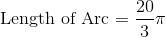# Intermediate Geometry : How to find the length of an arc

## Example Questions

### Example Question #81 : Plane Geometry

The radius of a circle is. Find the length of an arc if it has a measure ofdegrees.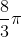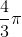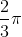Explanation:

Recall that the length of an arc is merely a part of the circle's circumference.

We can then write the following equation to find the length of an arc: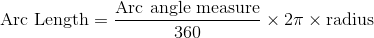Plug in the values of the arc angle measure and the radius to find the length of the arc.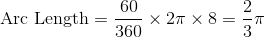### Example Question #82 : Plane Geometry

The radius of a circle is. Find the length of an arc if it has a measure ofdegrees.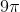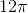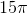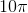Explanation:

Recall that the length of an arc is merely a part of the circle's circumference.

We can then write the following equation to find the length of an arc:Plug in the values of the arc angle measure and the radius to find the length of the arc.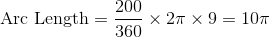### Example Question #83 : Plane Geometry

The radius of a circle is. Find the length of an arc if it has a measure ofdegrees.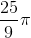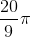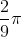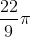Explanation:

Recall that the length of an arc is merely a part of the circle's circumference.

We can then write the following equation to find the length of an arc:Plug in the values of the arc angle measure and the radius to find the length of the arc.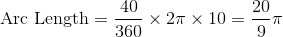### Example Question #84 : Plane Geometry

The radius of a circle is. Find the length of an arc if it has a measure of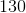degrees.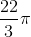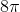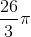Explanation:

Recall that the length of an arc is merely a part of the circle's circumference.

We can then write the following equation to find the length of an arc:Plug in the values of the arc angle measure and the radius to find the length of the arc.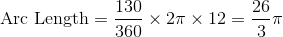### Example Question #85 : Plane Geometry

The radius of a circle is. Find the length of an arc if it has a measure ofdegrees.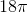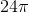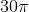Explanation:

Recall that the length of an arc is merely a part of the circle's circumference.

We can then write the following equation to find the length of an arc:Plug in the values of the arc angle measure and the radius to find the length of the arc.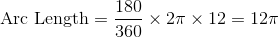### Example Question #86 : Plane Geometry

The radius of a circle is. Find the length of an arc if it has a measure of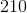degrees.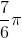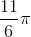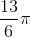Explanation:

Recall that the length of an arc is merely a part of the circle's circumference.

We can then write the following equation to find the length of an arc:Plug in the values of the arc angle measure and the radius to find the length of the arc.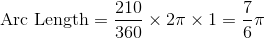### Example Question #87 : Plane Geometry

Find the length of the arc if the radius of a circle isand the measure of the central angle isdegrees.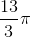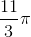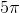Explanation:

An arc is just a piece—or a fraction—of a circle's circumference. Use the following formula to find the length of an arc: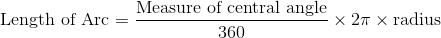Substitute in the given values for the central angle and the radius.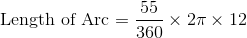Solve.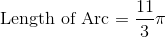### Example Question #88 : Plane Geometry

Find the length of an arc if the radius of the circle isand the measurement of the central angle isdegrees.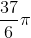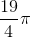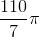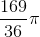Explanation:

An arc is just a piece—or a fraction—of a circle's circumference. Use the following formula to find the length of an arc:Substitute in the given values for the central angle and the radius.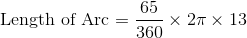Solve.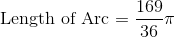### Example Question #89 : Plane Geometry

Find the length of an arc if the radius of the circle isand the measurement of the central angle isdegrees.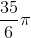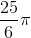Explanation:

An arc is just a piece—or a fraction—of a circle's circumference. Use the following formula to find the length of an arc:Substitute in the given values for the central angle and the radius.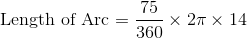Solve.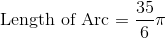### Example Question #90 : Plane Geometry

Find the length of an arc if the radius of the circle isand the measurement of the central angle isdegrees.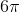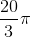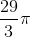Explanation:

An arc is just a piece—or a fraction—of a circle's circumference. Use the following formula to find the length of an arc:Substitute in the given values for the central angle and the radius.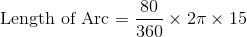Solve.